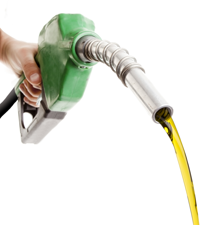# Applications

## Liquid DensityFuel adulteration can be detected using liquid density

Density is a fundamental property of all liquids. It is like a fingerprint for the substance. At a fixed temperature and pressure, a pure fluid will have a specific density. Density is thus an excellent technique for determining the purity of a substance. The correlation of measured density to the density water is called specific gravity.

Liquid density is very sensitive to a change in temperature. The measurement of density and temperature should be closely coupled. Integrated Sensing Systems (ISS) liquid density meters include an integrated temperature sensor fabricated within the microCoriolis sensor. For applications using a bypass flow, a remote temperature probe input is available to correct measured density to line conditions.

Integrated Sensing Systems liquid density meters can report measured density, density referenced to a specific temperature and specific gravity.

The applications for liquid density measurement in process monitoring and control are very extensive. Here are just a few applications for liquid density measurement.

• Correction of orifice flow measurement to liquid density changes
• Conversion of volumetric flow measurement to mass flow measurement
• Product quality determination
• Fuel adulteration detection

## Gas DensityHeating value of a fuel can be inferred using gas density

The density of gas is proportional to the gas molecular weight, pressure and temperature. Small changes in pressure can have a great impact on gas density. When reporting gas density it is important to correlate it to a measured gas pressure.

Integrated Sensing Systems includes a pressure sensor with every gas density meter. Specific gravity, reference density and average molecular weight of the gas can be determined based on the measured density, temperature and pressure.

The application of gas density for process monitoring and control is very extensive. Here are just a few applications for gas density measurement.

• Correction of orifice flow measurement to gas density changes
• Conversion of gas volume measurement to mass measurement
• Heating value estimation
• Gas mixing control

## Liquid Concentration MeasurementThe purity of alcohol can be determined using liquid specific gravity measurement

Liquid density measurement can be used to calculate the concentration of binary fluids containing two components of different pure fluid densities or a pure liquid containing dissolved solids. The Integrated Sensing Systems density meter can be programmed with a polynomial mathematical equation that converts the measured density and temperature into a composition. What is required is tabulated data of density, temperature and composition. The reliability of the density calculation is a result of the accuracy of the tabulated data and the accuracy of the temperature measurement. For measurement of small concentrations it is important to take into consideration the compressibility of the fluid. Fluids like alcohol have compressibilities of over 100 parts per million per 15 psi of pressure. For low concentration measurements such as trace water in ethanol, this should be taken into account. The Integrated Sensing Systems liquid density meters can either be programmed to the operating pressure of the system or can use the external pressure sensor for live corrections of pressure.

The specific gravity of a fluid is the comparison of either the measured density at the fluid temperature divided by the density of water at the same temperature. Specific gravity can also be expressed at a specific temperature if the expansion coefficient of the fluid is known.

These are examples of liquid concentration measurements that can be accomplished using liquid density measurements.

• Ethanol concentration in water
• Salt concentration in water
• Sugar concentration (BRIX)
• Glycol concentration in water

## Gas Concentration MeasurementBiogas methane concentration can be detected using average molecular weight calculation

Gas density measurement can be used to calculate the concentration of binary gas mixtures with gas component molecular weights that vary by at least 15. The Integrated Sensing Systems gas density meter can use either the ideal gas equation to calculate concentration or a polynomial mathematical equation that converts the measured density, pressure and temperature into a gas composition. Tabulated data of density, temperature and composition is required to use the equation.

Specific gravity of a gas is the comparison of the measured gas density to the gas density of air at the same temperature and pressure. The Integrated Sensing Systems gas density density meter will calculate specific gravity of a gas.

These are examples of gas blend concentrations that can be measured using the Integrated Sensing Systems gas density meter.

• Propane-air blend ratio measurement
• Hydrogen measurement in a gas mixture
• Forming gas blending
• Vapor concentration in a gas
• Methane concentration in biogas

## Low Liquid Mass Flow MeasurementLiquid Droplets

The Integrated Sensing Systems MassSense® (MS-LFV) is the next step in low flow liquid mass measurement technology by using a patented* microCoriolis™ fluidic sensor utilizing silicon MEMS technology. The traditional components of a steel Coriolis flow meter, are now fabricated using silicon processing technology. The result is a sensor that provides low mass flow measurement in a package smaller than a fingernail. Silicon’s low weight (1/3 that of stainless steel) and very high strength (3x that of stainless steel) makes it a perfect material to construct a low-flow Coriolis mass flowmeter.

A microCoriolis sensor is the perfect solution for low flow measurement in research, pilot plant and lab applications. Coriolis technology measurement accuracy is not dependent on knowledge of the thermal properties of the fluid. It measures mass flow directly. The small volume, silicon sensing tube, vibrates at high frequency >20kHz, eliminating the effect of external vibration. The mass flow of fluid causes a twist in the vibrating U shaped silicon tube, proportional to the mass flow. The MassSense® LFV has a very large dynamic measurement range, from 5 grams/hr to 1000 grams/hr with an accuracy of 0.15% of rate.

These are examples of applications where the Integrated Sensing Systems liquid flow meter can be used.

• CVD thin film deposition using liquid precursors
• Pilot plant low flow liquid metering
• Laboratory flow measurements
• Chemical dosing
• Metrology